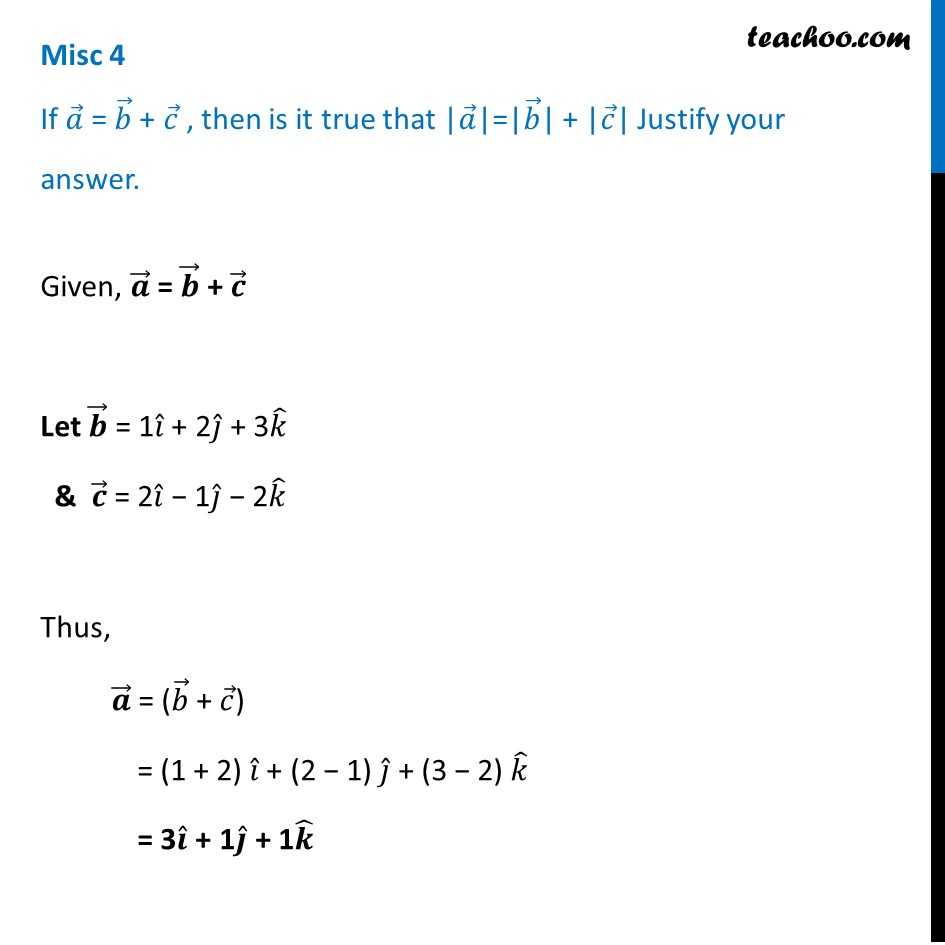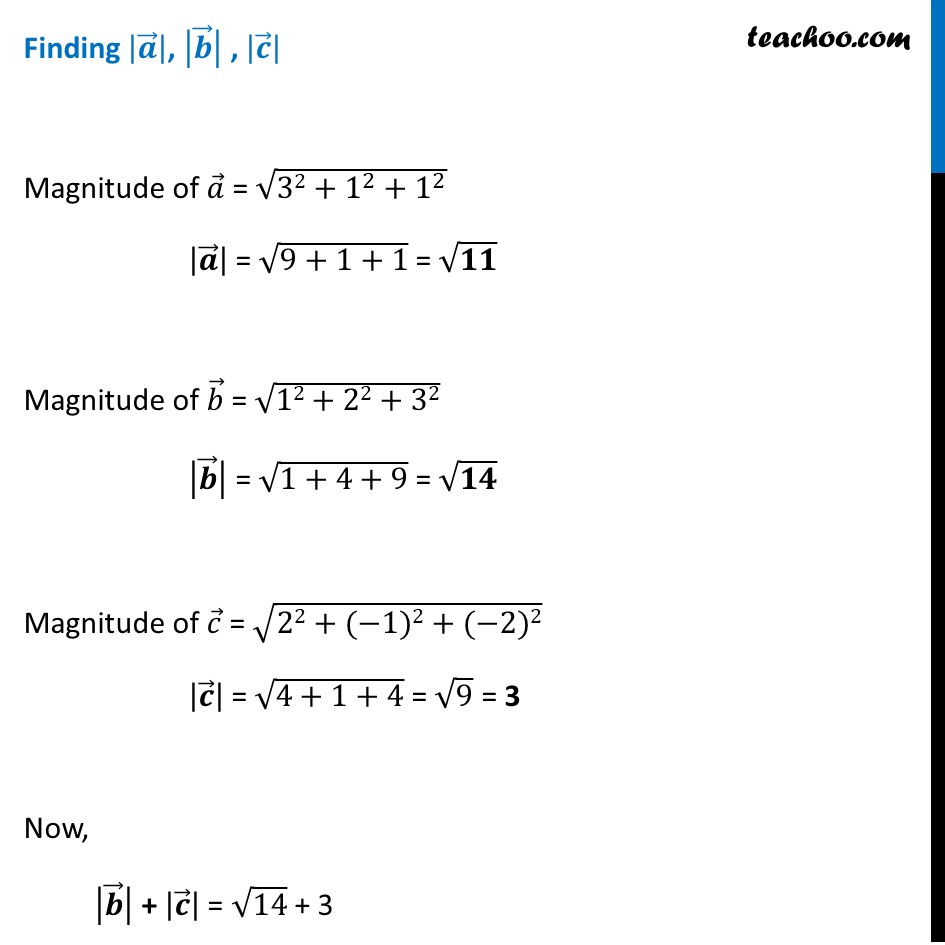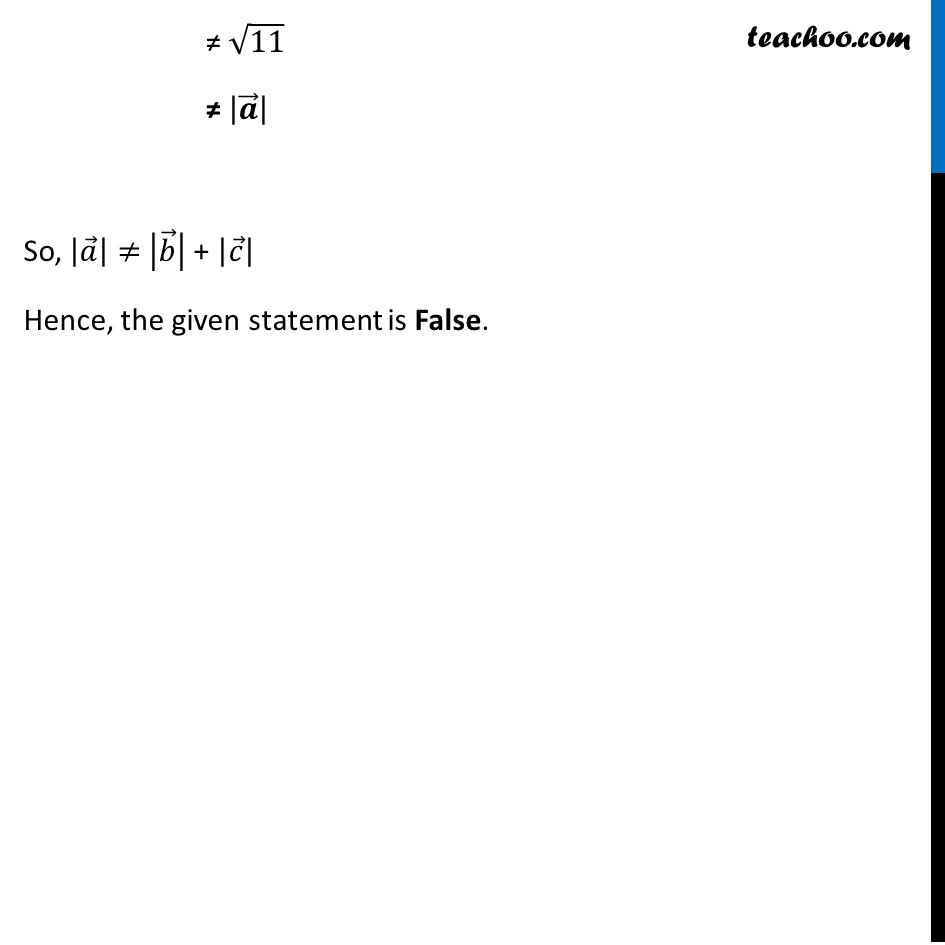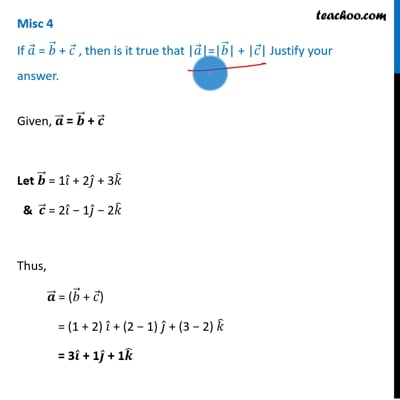Chapter 10 Class 12 Vector Algebra
Concept wiseThis video is only available for Teachoo black users

Introducing your new favourite teacher - Teachoo Black, at only ₹83 per month

### Transcript

Misc 4 If 𝑎 ⃗ = 𝑏 ⃗ + 𝑐 ⃗ , then is it true that |𝑎 ⃗|=|𝑏 ⃗| + |𝑐 ⃗| Justify your answer.Given, 𝒂 ⃗ = 𝒃 ⃗ + 𝒄 ⃗ Let 𝒃 ⃗ = 1𝑖 ̂ + 2𝑗 ̂ + 3𝑘 ̂ & 𝒄 ⃗ = 2𝑖 ̂ − 1𝑗 ̂ − 2𝑘 ̂ Thus, 𝒂 ⃗ = (𝑏 ⃗ + 𝑐 ⃗) = (1 + 2) 𝑖 ̂ + (2 − 1) 𝑗 ̂ + (3 − 2) 𝑘 ̂ = 3𝒊 ̂ + 1𝒋 ̂ + 1𝒌 ̂ Given, 𝒂 ⃗ = 𝒃 ⃗ + 𝒄 ⃗ Let 𝒃 ⃗ = 1𝑖 ̂ + 2𝑗 ̂ + 3𝑘 ̂ & 𝒄 ⃗ = 2𝑖 ̂ − 1𝑗 ̂ − 2𝑘 ̂ Thus, 𝒂 ⃗ = (𝑏 ⃗ + 𝑐 ⃗) = (1 + 2) 𝑖 ̂ + (2 − 1) 𝑗 ̂ + (3 − 2) 𝑘 ̂ = 3𝒊 ̂ + 1𝒋 ̂ + 1𝒌 ̂ Given, 𝒂 ⃗ = 𝒃 ⃗ + 𝒄 ⃗ Let 𝒃 ⃗ = 1𝑖 ̂ + 2𝑗 ̂ + 3𝑘 ̂ & 𝒄 ⃗ = 2𝑖 ̂ − 1𝑗 ̂ − 2𝑘 ̂ Thus, 𝒂 ⃗ = (𝑏 ⃗ + 𝑐 ⃗) = (1 + 2) 𝑖 ̂ + (2 − 1) 𝑗 ̂ + (3 − 2) 𝑘 ̂ = 3𝒊 ̂ + 1𝒋 ̂ + 1𝒌 ̂ Finding |𝒂 ⃗ |, |𝒃 ⃗ | , |𝒄 ⃗ | Magnitude of 𝑎 ⃗ = √(32+1^2+1^2 ) |𝒂 ⃗ | = √(9+1+1) = √𝟏𝟏 Magnitude of 𝑏 ⃗ = √(12+22+32) |𝒃 ⃗ | = √(1+4+9) = √𝟏𝟒 Magnitude of 𝑐 ⃗ = √(22+(−1)2+(−2)2) |𝒄 ⃗ | = √(4+1+4) = √9 = 3 Now, |𝒃 ⃗ | + |𝒄 ⃗ | = √14 + 3 ≠ √11 ≠ |𝒂 ⃗ | So, |𝑎 ⃗ |≠ |𝑏 ⃗ | + |𝑐 ⃗ | Hence, the given statement is False.